## On the Subject of Ultralogic

Wait a ████ing second... did we skip Hyperlogic?

• On the module, there are three squares labeled A, B, and C. There are also logic gates connecting A/B to C, B/C to A, and C/A to B. Green is “true”, and red is “false”.
• Clicking on the logic gates will change it to another. Before any spot is interacted with (it will be blank), you need to place down the operators by clicking on where they would be.
• When all three logic gates are set to a position that makes the logic correct, press the submit button.
• You cannot submit any duplicate operators.
 AND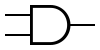If both inputs are true, the output is true. Otherwise, the output is false. OR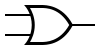If either input is true, the output is true.Otherwise, the output is false. XOR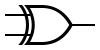If exactly one input is true, the output is true.Otherwise, the output is false. NAND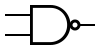If both inputs are true, the output is false.Otherwise, the output is true. NOR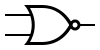If either input is true, the output is false.Otherwise, the output is true. XNOR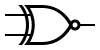If exactly one input is true, the output is false.Otherwise, the output is true.